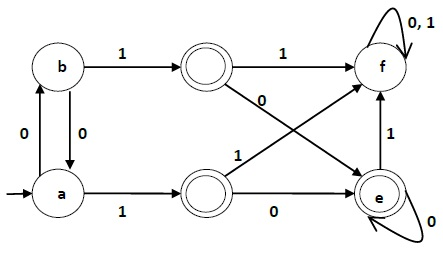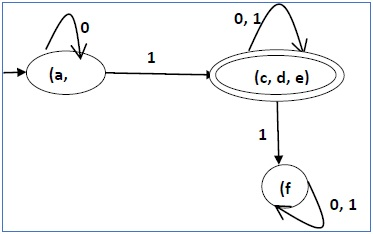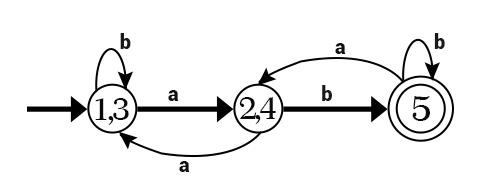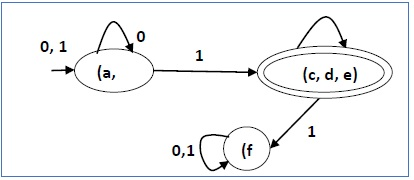# DFA Minimization

## DFA Minimization using Myphill-Nerode Theorem

### Algorithm

Input − DFA

Output − Minimized DFA

Step 1 − Draw a table for all pairs of states (Qi, Qj) not necessarily connected directly [All are unmarked initially]

Step 2 − Consider every state pair (Qi, Qj) in the DFA where Qi ∈ F and Qj ∉ F or vice versa and mark them. [Here F is the set of final states]

Step 3 − Repeat this step until we cannot mark anymore states −

If there is an unmarked pair (Qi, Qj), mark it if the pair {δ (Qi, A), δ (Qi, A)} is marked for some input alphabet.

Step 4 − Combine all the unmarked pair (Qi, Qj) and make them a single state in the reduced DFA.

### Example

Let us use Algorithm 2 to minimize the DFA shown below.Step 1 − We draw a table for all pair of states.

 a b c d e f a b c d e f

Step 2 − We mark the state pairs.

 a b c d e f a b c ✔ ✔ d ✔ ✔ e ✔ ✔ f ✔ ✔ ✔

Step 3 − We will try to mark the state pairs, with green colored check mark, transitively. If we input 1 to state ‘a’ and ‘f’, it will go to state ‘c’ and ‘f’ respectively. (c, f) is already marked, hence we will mark pair (a, f). Now, we input 1 to state ‘b’ and ‘f’; it will go to state ‘d’ and ‘f’ respectively. (d, f) is already marked, hence we will mark pair (b, f).

 a b c d e f a b c ✔ ✔ d ✔ ✔ e ✔ ✔ f ✔ ✔ ✔ ✔ ✔

After step 3, we have got state combinations {a, b} {c, d} {c, e} {d, e} that are unmarked.

We can recombine {c, d} {c, e} {d, e} into {c, d, e}

Hence we got two combined states as − {a, b} and {c, d, e}

So the final minimized DFA will contain three states {f}, {a, b} and {c, d, e}## DFA Minimization using Equivalence Theorem

If X and Y are two states in a DFA, we can combine these two states into {X, Y} if they are not distinguishable. Two states are distinguishable, if there is at least one string S, such that one of δ (X, S) and δ (Y, S) is accepting and another is not accepting. Hence, a DFA is minimal if and only if all the states are distinguishable.

### Algorithm 3

Step 1 − All the states Q are divided in two partitions − final states and non-final states and are denoted by P0. All the states in a partition are 0th equivalent. Take a counter k and initialize it with 0.

Step 2 − Increment k by 1. For each partition in Pk, divide the states in Pk into two partitions if they are k-distinguishable. Two states within this partition X and Y are k-distinguishable if there is an input S such that δ(X, S) and δ(Y, S) are (k-1)-distinguishable.

Step 3 − If Pk ≠ Pk-1, repeat Step 2, otherwise go to Step 4.

Step 4 − Combine kth equivalent sets and make them the new states of the reduced DFA.

### Example

Let us consider the following DFA −q δ(q,0) δ(q,1)
a b c
b a d
c e f
d e f
e e f
f f f

Let us apply the above algorithm to the above DFA −

• P0 = {(c,d,e), (a,b,f)}
• P1 = {(c,d,e), (a,b),(f)}
• P2 = {(c,d,e), (a,b),(f)}

Hence, P1 = P2.

There are three states in the reduced DFA. The reduced DFA is as follows −Q δ(q,0) δ(q,1)
(a, b) (a, b) (c,d,e)
(c,d,e) (c,d,e) (f)
(f) (f) (f)